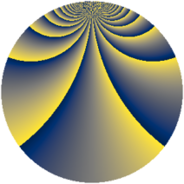# Properties

 Label 2300.2.bmLevel $2300$ Weight $2$ Character orbit 2300.bm Rep. character $\chi_{2300}(11,\cdot)$ Character field $\Q(\zeta_{110})$ Dimension $14240$ Sturm bound $720$

# Related objects

## Defining parameters

 Level: $$N$$ $$=$$ $$2300 = 2^{2} \cdot 5^{2} \cdot 23$$ Weight: $$k$$ $$=$$ $$2$$ Character orbit: $$[\chi]$$ $$=$$ 2300.bm (of order $$110$$ and degree $$40$$) Character conductor: $$\operatorname{cond}(\chi)$$ $$=$$ $$2300$$ Character field: $$\Q(\zeta_{110})$$ Sturm bound: $$720$$

## Dimensions

The following table gives the dimensions of various subspaces of $$M_{2}(2300, [\chi])$$.

Total New Old
Modular forms 14560 14560 0
Cusp forms 14240 14240 0
Eisenstein series 320 320 0

## Trace form

 $$14240q - 27q^{2} - 27q^{4} - 88q^{5} - 19q^{6} - 27q^{8} - 402q^{9} + O(q^{10})$$ $$14240q - 27q^{2} - 27q^{4} - 88q^{5} - 19q^{6} - 27q^{8} - 402q^{9} - 44q^{10} - 15q^{12} - 54q^{13} - 33q^{14} - 27q^{16} - 66q^{17} - 68q^{18} - 44q^{20} - 66q^{21} - 148q^{24} - 72q^{25} - 100q^{26} - 33q^{28} - 54q^{29} - 44q^{30} - 52q^{32} - 66q^{33} - 33q^{34} - 31q^{36} - 66q^{37} - 33q^{38} - 44q^{40} - 54q^{41} - 253q^{42} - 33q^{44} - 46q^{46} - 61q^{48} - 1472q^{49} + 68q^{50} + 173q^{52} - 66q^{53} - 139q^{54} - 33q^{56} - 176q^{57} - 67q^{58} - 44q^{60} - 66q^{61} + 47q^{62} - 27q^{64} - 88q^{65} + 33q^{66} - 72q^{69} - 64q^{70} + 22q^{72} - 54q^{73} - 88q^{74} + 22q^{76} - 18q^{77} - 139q^{78} + 55q^{80} + 510q^{81} - 33q^{84} - 176q^{85} - 363q^{86} - 33q^{88} - 66q^{89} + 143q^{90} + 83q^{92} - 216q^{93} - 83q^{94} + 337q^{96} - 66q^{97} - 15q^{98} + O(q^{100})$$

## Decomposition of $$S_{2}^{\mathrm{new}}(2300, [\chi])$$ into newform subspaces

The newforms in this space have not yet been added to the LMFDB.